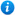* Watermarks do not appear on purchased resources

Preview resource: Click slides to enlarge

# Area - Year 5

Area - Year 5: KS2 Maths PowerPoint Activities

Year 5 maths programme of study - Measurement:

• calculate and compare the area of rectangles (including squares), and including using standard units, square centimetres (cm²) and square metres (m²) and estimate the area of irregular shapes

In this teaching resource, pupils practise measuring the area of rectangles and squares using a grid and the formula length x width and begin to measure the area of compound and irregular shapes. 'Area - Year 5' is an ideal teaching aid to use during a lesson covering the year 5 curriculum objective listed above and supports the White Rose small steps guidance for year 5 - Autumn - Block 5 - Perimeter and Area. Content includes:

• Counting 1 cm squares to find the area of rectangles and squares activity and worksheet
• Counting 1 cm squares to find the area of irregular shapes activity and worksheet
• Calculating area formula slide
• Calculating the area of squares and rectangles activity and worksheet
• Calculating the area of compound shapes activity and worksheet and 1 further differentiated worksheet
• Estimating the area of irregular shapes on a grid slide
• Estimating the area of irregular shapes activity and worksheet
• Four reasoning and problem solving activities and accompanying worksheets

This animated maths PowerPoint presentation can be edited so teachers are able to adapt the resource to suit their individual teaching requirements.

Preview 'Area - Year 5' in more detail by clicking on the PowerPoint images.

Our Price : £3.99 / 4 Credits